Journal of Global Change Data & Discovery2022.6(4):509-520

Citation：Dai, X. L., Zhu, Y. Q., Yang, J., et al.Research and Implementation of Geospatial Data Similarity Calculation Method[J]. Journal of Global Change Data & Discovery,2022.6(4):509-520 .DOI: 10.3974/geodp.2022.04.01 .

# Research and Implementation of Geospatial Data Similarity Calculation Method

## Dai, X. L.1,2  Zhu, Y. Q.1,3*  Yang, J.1  Sun, K.1  Li, J. D.4  Song, J.1,3

1. State Key Laboratory of Resources and Environmental Information System, Institute of Geographic Sciences and Natural Resources Research, Chinese Academy of Sciences, Beijing 100101, China;

2. University of Chinese Academy of Sciences, Beijing 100049, China;

3. Jiangsu Center for Collaborative Innovation in Geographical Information Resource Development and Application, Nanjing 210023, China;

4. Dongying Ecology and Environment Bureau, Dongying 257091, China

Abstract: Geospatial data similarity calculation is one of the key technologies for intelligent data recommendation and discovery. Existing methods for geospatial data similarity calculation can be classified into methods based on file information, metadata, or dataset. Each of these methods has advantages and disadvantages. Nevertheless, problems such as inaccurate calculation results or extensive calculation due to missing information items may frequently occur if only one type of information is used for similarity calculation, thus affecting the application value of data similarity. To give full play to the advantages of various methods, this paper proposes a geospatial data similarity calculation method integrating three levels of file information, metadata, and dataset, and develops a corresponding software, which can effectively improve the calculation accuracy and efficiency of geospatial data similarity according to its actual situation.

Keywords: geospatial data; data similarity; calculation method; software

DOI: https://doi.org/10.3974/geodp.2022.04.01

CSTR: https://cstr.escience.org.cn/CSTR:20146.14.2022.04.01

Dataset Availability Statement:

The dataset supporting this paper was published and is accessible through the Digital Journal of Global Change Data Repository at: https://doi.org/10.3974/geodb.2022.10.02.V1 or https://cstr.escience.org.cn/CSTR:20146.11.2022.10.02.V1.

### 1 Introduction

Geospatial data is a digital representation of the spatial and attribute characteristics of geographic entities and phenomena, and is one of the basic elements of geoscientific research . Geospatial data plays an important role in natural and social science research and has been widely used in many fields such as emergency management , environmental monitoring , natural disaster prediction , and population economic research . Geospatial data similarity calculation is to calculate the similarity between data based on their features. It is one of the key technologies of data intelligent recommendation and discovery and is widely used in the processes of geospatial data association network , geospatial data intelligent recommendation , and geospatial model automatic matching data . In addition, analogous to text similarity used for document duplication checking, geospatial data similarity calculation can also be used for geospatial data duplication checking.

According to the sources of data feature information used for similarity calculation, the existing geospatial data similarity calculation methods are divided into three categories: (1) similarity calculation methods based on file information. This method mainly calculates the data similarity by information items of data files, such as file name, file format, etc. For example, Sun et al. proposed a similarity calculation model for P2P file sharing applications using file information such as file name, file length, and file type ; Kim used a function matching approach for similarity calculation of binary files ; Kim et al. proposed a partial hash information string algorithm for similarity calculation of multimedia data files . Such methods are computationally simple and efficient, yet they can only be used to calculate fewer data features, and inaccurate recording of file information may occur (e.g., file names are modified). (2) Metadata-based similarity calculation method. This method mainly calculates data similarity by metadata items, such as content topic, time range, spatial range, etc. For example, Zhu et al. calculated geographic metadata similarity based on eight data attributes such as data topic, category, spatial coverage, temporal coverage, data type, and data format to quantitatively interconnect geographic data ; Chen et al. used artificial neural networks to calculate geographic metadata similarity based on data attributes of keywords, category, spatial coverage, and temporal coverage of data, and then recommended geographic data based on the similarity of metadata . These methods enable a more comprehensive similarity calculation with abundant metadata, yet sometimes there are many uncertainties, such as missing metadata, incomplete and imprecise records (e.g., the minimum outer rectangle cannot fully express the data range of the space). (3) Similarity calculation method based on dataset. This method mainly calculates the data similarity through the element location and attribute information of the dataset. For example, Mei et al. established a calculation model of point feature similarity by studying the similarity of density, area, and spatial direction of point groups ; Zhu et al. calculated the similarity of grid images based on the similarity relationship of color histograms . By using element location and attribute information of data entities, these methods can fully reflect the similarities in the content of data entities. Nevertheless, some information items (e.g., temporal information of vector data) are not directly reflected in the data entities and are computationally intensive as they are calculated either element by element or image by image.

Each of the above three types of methods has its own advantages and disadvantages, and most of the current studies use only one of them, which are either not very accurate or computationally intensive and time-consuming; in addition, the problem that the similarity cannot be calculated due to the lack of necessary feature factors occurs. This paper proposes a method for calculating the similarity of geospatial data that integrates file information, metadata, and dataset. It can comprehensively use the advantages of each method, and flexibly select different methods and their combinations according to data information and practical application requirements, to achieve fast and accurate data similarity calculation. In addition, by comparing the similarity calculation results of the three levels, it is beneficial to discover the differences between the data. To realize the calculation of geospatial similarity, the corresponding software was developed to accompany it.

### 2 Geospatial Data Similarity Calculation

2.1 Similarity Factors Selection

The selection of a suitable similarity factor is a prerequisite for similarity calculation. By analyzing the characteristics of the three levels, the corresponding similarity factors were selected.

The information items used for file information similarity calculation mainly include file name, file format, file size, number of files, and the like. A file name is a name that identifies one piece of data from another. Different data usually have different data formats. The same data usually has the same file size and quantity.

Geospatial metadata is used to describe the geospatial data and  mainly includes information about the content topics, temporal scope and spatial extent of geospatial data. There are many different metadata standards, such as ISO19115 metadata standard for geographic information , Content Standard for Digital Geospatial Metadata (CSDGM) , Geographic Information Metadata (GB/T19710--2005), etc. Most of the metadata standards include metadata items such as content topic, spatial extent, temporal extent, spatial precision, temporal granularity, etc. Hence, this paper selects the above metadata items to calculate the metadata similarity.

Geospatial dataset mainly includes two types of data features: entity feature location and attribute. The feature of entity feature location is the location information of geospatial dataset on pixels or graphics. The feature of entity feature attribute refers to the attribute items and attribute values contained in the attribute table. Geospatial dataset formats mainly include raster and vector types. The similarity of raster dataset is mainly calculated based on image elements; as vector data can be further divided into point elements, line elements and surface elements, their similarity calculation needs to be expanded by point, line, and surface elements respectively. Among them, the location similarity of entity elements of point elements is mainly based on point group topology, distribution range, direction relationship and distance for similarity calculation, and the location similarity of entity elements of line and area elements is mainly based on the topological relationship and distance of line or area groups. Relationships, directional relationships, and geometric features are calculated.

2.2 Overall Calculation Process

The overall flow of the integrated calculation method of geospatial data similarity is shown in Figure 1. The basic steps of the algorithm are: (1) First, calculate the file information similarity. Using this method to calculate data similarity is simple and fast, but the similarity obtained is coarse grained, not accurate enough; (2) Then the metadata similarity is further calculated. This method presupposes the availability of metadata, moderate calculation and medium granularity of similarity, but depends on the quality of metadata, which may be missing the metadata or inaccurately described; (3) Finally, the dataset similarity is further calculated. The data entity similarity has high precision, and the obtained similarity is fine-grained, but the computation is heavy.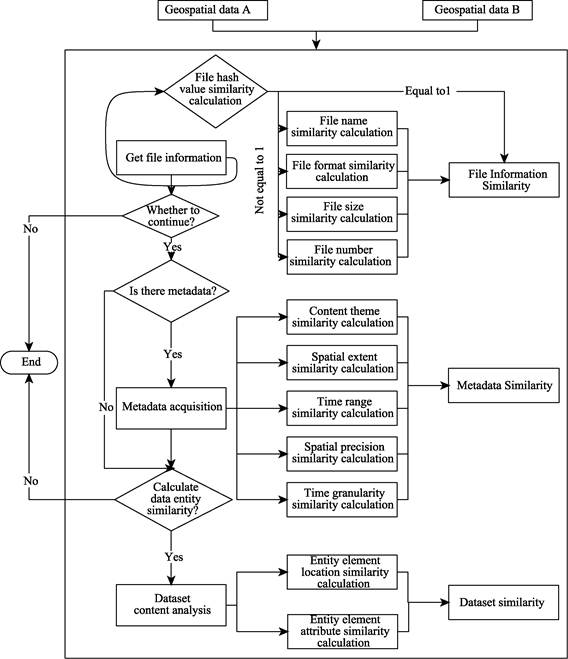Figure 1  The overall flow of the integrated calculation method of geospatial data similarity

2.3 Similarity Calculation Method

2.3.1 File Information Similarity Calculation Method

(1) Calculation method of file hash value similarity

File hash is a unique string generated with a hashing algorithm based on file information such as file name, size, format, etc. Files with identical file information will generate the same hash value under the same hashing algorithm, but with minor differences in file information, their hash values will vary greatly. Therefore, the file hash value (Shash) can quickly determine whether the file information is the same. In this paper, we use sha256 hash algorithm  to generate data file hash, when two hash values are the same, then the file hash similarity is 1, otherwise the hash similarity is null (None), and we need to continue to calculate the similarity of file name, format, size, and number.

(2) Calculation method of file name similarity

The text of the file name is short, and a string-based semantic text similarity algorithm is usually used to judge the consistency of the file name. By analyzing the characteristics of commonly used string-based text similarity calculation methods, this paper selects the Minimum Edit Distance (MED) algorithm  for file name similarity calculation.

Let UA and UB be the file names of the two data,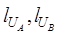are the lengths of the two file names respectively, that is, the number of characters in the string, and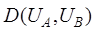is the edit distance of the file names A and B, then the file name similarity of geospatial data is calculated using Equation (1) .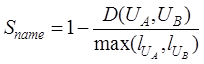(1)

(3) Calculation method of file format similarity

Geospatial data formats mainly include raster and vector formats. The similarity of data formats depends on the difficulty of converting between the two types of data. The easier the data conversion, the higher the similarity of the data format of the two data . According to the difficulty of data conversion, this paper divides the similarity calculation of data formats into three cases: the same data format, the data format of the same group and the data format of different groups.

If the data formats of the two data are the same, no data conversion is required, so the data format similarity is 1. The data format of the same family refers to the series of formats that can be supported by the software products of the same company (such as the data formats supported by ArcGIS products, shapefile, E00, etc.), whose data conversion can be relatively easy to achieve through the existing data processing tools. It has been studied that the data similarity of data formats of the same family is set to 0.85 .

The difficulty of converting different families of data formats is much more complicated compared to the two formats, and its conversion difficulty is determined by the openness of the data formats, and the specific similarity calculation method can be found in the literature of Zhu et al. (2017) .

(4) Calculation method of file number similarity

The number of files of data is an integer type value greater than 0. Calculating the similarity of the number of files of geospatial data only requires comparing the size of the number of files of two geospatial data. Therefore, the similarity of the number of files of geospatial data is calculated by Equation (2).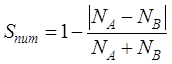(2)

where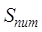is the similarity between the number of files of dataset A and dataset B, and NA and NB are the number of files of dataset A and dataset B, respectively.

(5) Calculation method of file size similarity

File size is the amount of data stored in the computer and is the sum of the sizes of all files that make up geospatial data. Since different compression methods change the file size, the file size in this paper is the size of the file in its uncompressed state.

Calculating the file size similarity of geospatial data requires converting the size of all files of both geospatial data to a value under the same unit, such as a uniform KB or MB. The storage size similarity of geospatial data can be calculated by equation (3).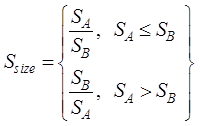(3)

Where,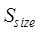is the similarity between the file sizes of dataset A and dataset B, and SA and SB are the file sizes of dataset A and dataset B, respectively.

(1) Calculation method of content theme similarity

The content theme features of geospatial data mainly consist of metadata titles, keywords and abstracts. The content topic similarity can be calculated based on the subject terms of these metadata items. Therefore, the content topic similarity of geospatial data can be calculated by equation (4).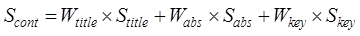(4)

Where,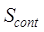is the content subject similarity,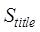is the title similarity,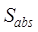is the summary similarity,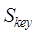is the keyword similarity, and, W, W and W are the weights of title, summary and keyword respectively. According to Zhu et al. (2017) literature , the weight of title, keyword and abstract can be set to 0.529, 0.309 and 0.162 respectively.

The similarity of titles, keywords, and abstracts is measured by the similarity of the corresponding keywords, respectively. The calculation process of the similarity of the subject words in this paper is as follows: (1) Use the word segmentation tool to divide the continuous text in the extracted title and abstract into multiple words; (2) Remove the stop words that are meaningless for similarity calculation, such as "De"(of) and "de"(to) in Chinese, articles in English, etc., and then get the subject heading; (3) Calculate the feature weight through the term frequency-inverse document frequency (TF-IDF), which vectorizes subject terms separately; (4) Use the cosine similarity to calculate the similarity of the subject terms.

(2) Calculation method of spatial extent similarity

The spatial extent of geospatial data is usually represented by the Minimum Bounding Rectangle (MBR) of the dataset. Therefore, in this paper, the spatial extent of geospatial data is represented by the MBR. The data spatial extent topological relationship is first calculated, and then its spatial metric relationship is further calculated as shown in Equation (5).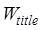(5)

Among them: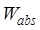is the spatial range similarity,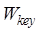and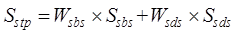are the spatial topological relationship similarity and spatial metric relationship similarity respectively,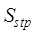and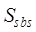are the corresponding weights, and the specific similarity calculation method and weight determination method refer to Zhu et al. (2017) Literature .

(3) Calculation method of time range similarity

Time of geospatial data usually has two forms: instantaneous time (time point) and period (time range). A time horizon usually consists of two time points: the start time and the end time. Instantaneous time and period are relative and can be interconverted at different time scales.

According to the previous analysis, there are three situations in the time range similarity calculation of geospatial data: (1) Both times are instantaneous; (2) One time is instantaneous time, the other is time end; (3) Both times are time periods. For the second case, the instantaneous time can be converted into time interval by time downscaling method, and the two-time intervals can be unified into the minimum time scale. For example, if the time scale of one geospatial data is "year" (2020) and the time scale of another geospatial data is "month" (March 2020 - March 2021), then it is necessary to convert the point time with the time scale of "year" (2020) to the time period with the time scale of "month" (January 2020 - December 2020), so as to keep the two geospatial data with a unified time scale. From this, the similarity of their time ranges can be calculated. Therefore, the three cases of time range similarity calculation of two kinds of geospatial data can be converted to time interval under the same time scale for calculation.

The time range similarity of geospatial data can be calculated by combining temporal topological, sequential and measurement relationships . Temporal topological relation is the temporal relation of geographical phenomena, representing the time when one geospatial data is between, after or simultaneous with another one. In the case of simultaneous time (equal or intersecting), the sequence of two-time ranges is also considered. The time measurement relationship includes two indicators: time overlap ratio and time distance. In addition, time sequence is also an important indicator to be considered when calculating time range similarity. It is generally assumed that new data are better than old data. Therefore, the time range similarity of geospatial data in this paper is calculated by Equation (6):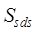(6)

Where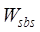is the temporal range similarity,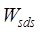and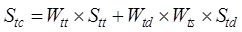are the temporal topological similarity and temporal distance similarity, respectively,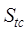and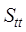are the weights of temporal topological relations and temporal metric relations, respectively, and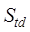is the temporal order degree, whose specific similarity calculation method and weight determination method refer to Chen et al. (2018) literature .

(4) Calculation method of spatial precision similarity

The spatial accuracy of geospatial data is usually expressed by the spatial scale (vector)/resolution (raster) and spatial granularity. The similarity of spatial accuracy of geospatial data is calculated by equation (7):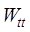(7)

Where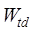denotes the spatial precision similarity;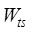and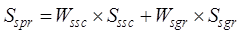denote the similarity of spatial scale (resolution) and spatial granularity, respectively;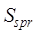and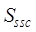are the corresponding weights Its specific similarity calculation method and weight determination method refer to Zhu et al. (2017) literature .

(5) Calculation method of time granularity similarity

The time granularity of geospatial data is generally represented by the data update frequency. For example, the update interval of the land cover data GlobeLand30 is 10 years. Time granularity is generally measured by the difficulty of transformation. Different time granularities can be transformed by the time scale up or down extrapolation method. Upward extrapolation refers to changing the finer time granularity into the coarser time granularity, which makes the expression process of geographical entities and phenomena sketchier; downward extrapolation is the opposite. When the time granularity of two geospatial data is the same, fine-coarse and coarse-fine, the similarity of time granularity is 1, 0.875 and 0.125  respectively.

2.3.3 Dataset Similarity Method

(1) Calculation method of location similarity of entity elements

Dataset similarity is a similarity calculation performed at the level of data entity elements. The premise is that the data types of the data sets to be calculated must be the same, such as vector data or raster data. The location similarity of entity elements of raster data is mainly calculated based on the overlapping area of ​​two raster data. Before calculating the similarity, the coordinate system and resolution of the two-raster data are unified, and then calculated according to Equation (8):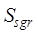(8)

where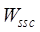is the similarity of entity element locations of raster data,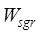and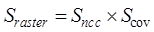are the normalized correlation coefficients and overlap ratios of the overlapping regions of the two raster data, respectively.

For the normalization coefficient of the overlapping area of ​​the raster data, the Equation (9) is used to calculate :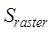(9)

where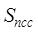and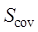are the image values of raster data A and B with ranks iand j, respectively,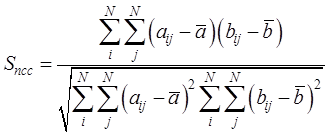and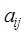are the average values of image elements in the overlapping area of raster data A and B, respectively, and N is the number of image elements in the area.

For the overlap ratio of two raster data, use Equation (10) to calculate: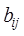(10)

where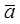is the overlapping area of raster data A and B, and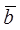,are the areas of raster data A and B, respectively.

The location similarity of entity elements of vector elements is calculated in three cases: point elements, line elements and polygon elements.

The location similarity of entity elements of point element data is determined by the topological relationship, distance relationship, directional relationship, distribution range and density between point groups, and is calculated by Equation (11):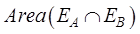(11)

where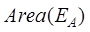is the location similarity of entity elements of point element data,,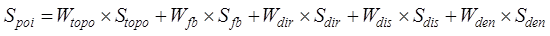,,and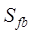are the topological similarity, distribution range similarity, directional relationship similarity, distance relationship similarity and distribution density similarity of point element data, respectively, and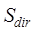,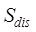,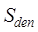,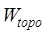and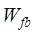are their corresponding weights, and the specific calculation methods and corresponding weights of each similarity of the above point elements are referred to the literature .

The location similarity of the entity elements of the line element data is determined by the topological relationship, distance relationship, direction relationship and geometric characteristics between the line groups, and is calculated by Equation (12):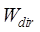(12)

where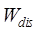is the solid element location similarity of the line element data,,,andare the topological similarity, directional relationship similarity, distance relationship similarity and geometric feature similarity of the line element data, respectively, and W , W , W and W are their corresponding weights, and the specific calculation methods and corresponding weights of each similarity of the above line elements are referred to the literature .

The location similarity of solid elements for the face element data is calculated in the same way as for the line elements, and the specific calculation method and corresponding weights for each similarity of the face elements are referred to the literature .

(2) Calculation method of attribute similarity of entity elements

The attributes of geospatial data are usually represented by the attribute item names and attribute values in the attribute table of the layer, so the attribute similarity is usually determined by the attribute item names and attribute values, which is calculated as shown in Equation (13).(13)

Where,and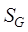are the similarity of attribute item names and attribute values, respectively, and W and W are their corresponding weights. According to reference , the values of W and W are 0.4 and 0.6, respectively. The similarity of both attribute item names, and attribute values is measured overall by the edit distance algorithm.

2.3.4 Similarity Aggregation Method and Application Strategy

(1) Similarity aggregation method

Based on the similarity of the above single feature or metadata item, the hierarchical analysis method can be used to calculate the composite similarity of each level (file similarity, metadata similarity and dataset similarity). The specific method is shown in Equation (14).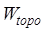(14)

where S is the composite similarity of each level, Si and Wi are the i-th single feature similarity and the corresponding weights, n represents the number of single feature similarities, and Wi are calculated using hierarchical analysis (Table 1). When a single similarity is missing, the other similarities at that level are reassigned with local weights according to the similarity size of the local weights for the composite similarity of that level.

Table 1  Weights for calculating the composite similarity at each level

 Compound similarity Single similarity Local weights File information similarity File name similarity 0.500 File format similarity 0.200 File size similarity 0.200 File number similarity 0.100 Metadata Similarity Content theme similarity 0.550 Spatial extent similarity 0.100 Time range similarity 0.150 Spatial precision similarity 0.100 Time granularity similarity 0.100 Dataset similarity Location similarity of entity elements 0.625 Attribute similarity of entity elements 0.375

(2) Application strategy

Firstly, the similarity of the two data is roughly calculated by the file information similarity, and then the metadata similarity is calculated. If the metadata is missing or a  more accurate similarity is required, the dataset similarity can be further calculated, thus forming different levels of data similarity calculation to meet the application requirements and achieve the objectives of both accuracy and efficiency of geospatial data confidence calculation.

### 3 Systems Software

3.1 Geospatial Data Similarity Calculation Software

To facilitate the understanding and application of geospatial data similarity calculation, this paper developed Geospatial Data Similarity Calculation Software (GDSCS V1.0) based on Python language . Due to the complexity and diversity of geospatial data, this software is implemented only using the National Tibetan Plateau Data Center as the data source.

3.2 GDSCS Software Functions and Test Results

GDSCS is a geospatial similarity computing software which integrates feature analysis, similarity calculation and result visualization based on geospatial data similarity calculation method. The functions of the software include geospatial data input, data feature information extraction, data similarity calculation, visualization, and derivation of the results. “Vegetation coverage data of Sichuan-Tibet traffic corridor (1985-2020) ” (hereinafter referred to as Data A) and “Vegetation cover resilience data set for countries along the Belt and Road (2000-2020) ” (hereinafter referred to as Data B) were randomly selected from the National Qinghai-Tibet Plateau Data Center as experimental data to test the operation and results of the GDSCS system. The file information of data A and data B is shown in Table 3.

The results of the file test, metadata test and entity data test using GDSCS software are shown in Figure 2, 3 and 4. It should be noted that the metadata input format is json, and the entity data input format are vector(.shp)and raster(.tiff).

Table 3  File information of data A and data B

 File information item Data A Data B File name CZLD_VFC_1000m_2016-2020 vegetation_country File format tif shp File size 37.6MB 616MB File number 8 10Figure 2  The result of file information similarity calculationFigure 3  The result of metadata similarity calculation resultsFigure 4  The result of dataset similarity calculation results

### 4 Discussion and Conclusion

Geospatial data similarity calculation and application is of great value and significance for geospatial data sharing and intelligent discovery. The GDSCS method proposed in this paper provides an operational tool for similarity calculation and dataset checking of scientific data and lays the foundation for further improvement. GDSCSV1 has further aspects of improvement in both theoretical methods and tool implementation. For example, in the calculation of file information similarity, the degree of file information association, the diversity of metadata formats, the diversity of data formats of entity datasets, the content association of spatial data, and the particularity of similarity calculation of systematic spatial location offset need to be further improved and perfected.

Author Contributions

Zhu, Y.Q. was responsible for the overall design of the method and the revision of the paper; Dai, X. L. carried out the method practice and writing; Yang, J. carried out the method research; Sun, K. was responsible for the method research; Li, J.D. participated in the design of the method and the revision of the paper; Song, J. revised the paper.

Conflicts of Interest

The authors declare no conflicts of interest.

### References

       Zhu, Y., Yang, J. Automatic data matching for geospatial models: a new paradigm for geospatial data and models sharing [J]. Annals of GIS, 2019, 25(4): 283‒298.

       Chen, Z., Yang, Y. Semantic relatedness algorithm for keyword sets of geographic metadata [J]. Cartography and Geographic Information Science, 2020, 47(2): 125‒140.

       Chen, Z., Song, J., Yang, Y. An approach to measuring semantic relatedness of geographic terminologies using a thesaurus and lexical database sources[J]. ISPRS International Journal of Geo-Information, 2018, 7(3): 98.

       Zhao, H., Zhu, Y., Yang, H., et al. The semantic relevancy computation model on essential features of geospatial data[J]. Geographical Research, 2016, 35: 58‒70.

       Guo, H., Liu, Z., Jiang, H., et al. Big Earth Data: A new challenge and opportunity for Digital Earth’s development[J]. International Journal of Digital Earth, 2017, 10(1): 1‒12.

       Zhu, Y., Zhu, A.X., Song, J., et al. Multidimensional and quantitative interlinking approach for linked geospatial data[J]. International Journal of Digital Earth, 2017, 10(9): 923‒943.

       Boubenia, M., Belkhir, A., Bouyakoub, F.M. Combining linked open data similarity and relatedness for cross OSN recommendation[J]. International Journal on Semantic Web and Information Systems (IJSWIS), 2020, 16(2): 59‒90.

       Zhu, Y., Zhu, A.X., Feng, M., et al. A similarity-based automatic data recommendation approach for geographic models[J]. International Journal of Geographical Information Science, 2017, 31(7): 1403‒1424.

       Sun, J.J., Yu, J.G., Liu, X.T. Similarity-computing model for P2P file-sharing networks[J]. Computer Engineering and Applications, 2012, 48(4): 111‒114.

    Kim, T.G., Lee, Y.R., Kang, B.J., et al. Binary executable file similarity calculation using function matching[J]. The Journal of Supercomputing, 2019, 75(2): 607‒622.

    Kim, B.K., Oh, S.J., Jang, S.B., et al. File similarity evaluation scheme for multimedia data using partial hash information[J]. Multimedia Tools and Applications, 2017, 76(19): 19649‒19663.

    Liu, T., Du, Q.Y., Mao, H.C. Spatial similarity assessment model and its application in line groups[J]. Geomatics and Information Science of Wuhan University, 2012, 37(8): 992‒995.

    Zhu, S. Image Similarity Computation by Using Histogram Method[J]. Bulletin of Surveying and Mapping, 2018, 0(12): 96‒100.

    Karschnick, O., Kruse, F.A, Töpker, S., et al. The UDK and ISO 19115 Standard[C]. EnviroInfo, 2003: 475‒481.

    Authority, T.V. Content Standard for Digital Geospatial Metadata[D]. National Aeronautics and Space Administration, 1998.

    Jiang, J., Liu, R. China Geographic Information–Metadata GB/T 19710-2005[M]. World Spatial Metadata Standards: Elsevier Science, 2005.

    Rachmawati, D., Tarigan, J.T., Ginting A B C. A comparative study of Message Digest 5 (MD5) and SHA256 algorithm[C]. Journal of Physics: Conference Series. IOP Publishing, 2018, 978(1): 012116.

    Strube, M., Rapp, S., Müller, C. The influence of minimum edit distance on reference resolution[C]. Proceedings of the 2002 Conference on Empirical Methods in Natural Language Processing (EMNLP 2002). 2002: 312‒319.

    Zhao, Z.P., Yin, Z.M., Wang, Q.P., et al. An improved algorithm of Levenshtein Distance and its application in data processing[J]. Journal of computer applications, 2009, 29(2): 424‒426.

    Chen, Z., Song, J., Yang, Y.. Similarity measurement of metadata of geospatial data: An artificial neural network approach[J]. ISPRS International Journal of Geo-Information, 2018, 7(3): 90.

    Rao, Y.R., Prathapani, N., Nagabhooshanam, E. Application of normalized cross correlation to image registration[J]. International Journal of Research in Engineering and Technology, 2014, 3(5): 12‒16.

    Duan, X.Q., Liu, T., Wu, D. Spatial similarity assessment of point clusters in multi-scale map spaces based on analytic hierarchy process[J]. Journal of Geo-information Science, 2016, 18(10):1312‒1321.

    Liu, C., Yan, G.W. Geometry similarity assessment model of spatial polygon groups[J]. Journal of Geo- Information Science, 2013, 15(5): 635‒642.

    Tan. Y.B., Tang, Y., Li, X.L., et al. Semantic-based geographic feature property similarity measurement model [J]. Remote Sensing Information, 2017, 32(1): 126‒133.

    Dai, X.L., Zhu, Y.Q., Yang, J., et al. A Software for Geospatial Data Similarity Calculation [J/DB/OL]. Digital Journal of Global Change Data Repository, 2022. https://doi.org/10.3974/geodb.2022.10.02.V1.

    Sui, T. Vegetation coverage data of Sichuan Tibet traffic corridor (1985-2020) [OL]. National Tibetan Plateau Data Center, 2021. https://doi.org/10.11888/Soil.tpdc.271618.

    Xu, X. Vegetation cover resilience data set for countries along the Belt and Road (2000-2020) [OL]. National Tibetan Plateau Data Center, 2022. https://doi.org/10.11888/HumanNat.tpdc.272282.

 https://data.tpdc.ac.cn/